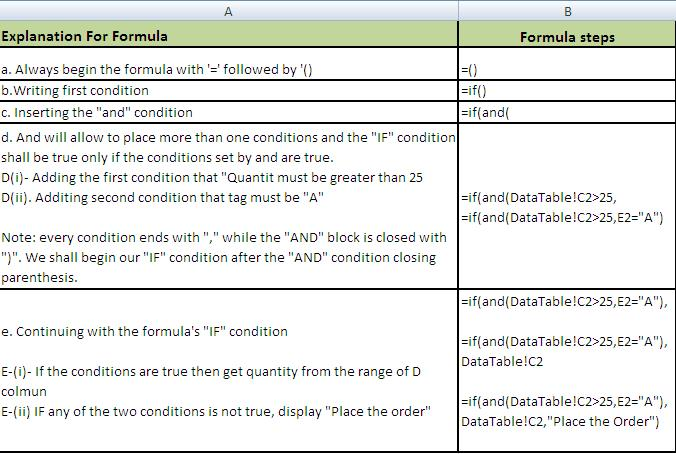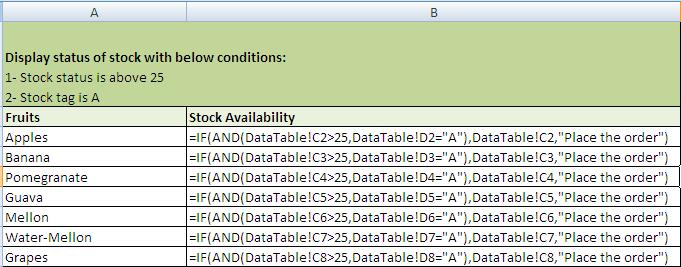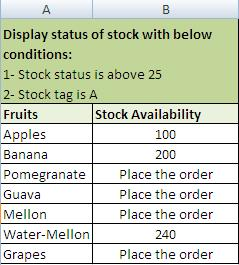# MS Excel Using IF AND Nested If Function

## Step by Step: How to Use Nested IF and ‘IF / And’ combination in excel 2003, 2007 & 2010

MS Excel Using IF AND Nested If Function: What is Nested IF and Use of AND in combination of IF Condition? Generally the IF() formula is used to specify a certain condition and if that condition is fulfilled, we can perform calculations when the criteria/condition meets or does not meet. It means that we have the option to output different results depending upon the result of IF condition. But IF condition can place only a single condition and there are situations where we need multiple criteria for display of results.

Here comes the use of AND() that allows to apply more than one conditions: an example is placed below:
A) Simple IF condition: =IF(A1=100, Value if true, values if false)
B) IF condition with And: =IF(AND(A1=100,B1>50,C1<10),Value if True, Value if False) The above underlines and italicized portion is for AND function which allows IF function to use more than one condition generally allowed. A detailed use of IF…AND Function in MS Excel 2007, 2010 is displayed below step by step:

### Step 1:

(b) Formulate your tables: these may be on the same table or on a different worksheet or even different workbooks.
(c) For the sake of example we have created two Tables.
(d) Table from where data is to be retrieved:IF AND MS Excel Formula-1

(e) Table where data is to be fetched/desired.IF AND MS EXCEL Formula 2

### Step 2:

Ensure spellings for search values are the same in both the tables.

### Step 3:

Make sure there is no extra space.

### Step 4:IF and MS Excel Function formula 3

### Final Formula:IF and MS Excel Formula 4

### Result:MS Excel IF and function-result

### Advantages of IF…AND (Nested If) formula:

1. You may copy and paste the formula anywhere within the same column but for this you must place dollar sign against table to lock it.
2. A really swift and smooth process for finding and counting of entries across the sheets and workbooks with ease and perfection.

### Things to Avoid:

1. If manually writing conditions on values in cells every time; make sure they are picked from drop down instead of manual entry.
2. Avoid extra spaces.